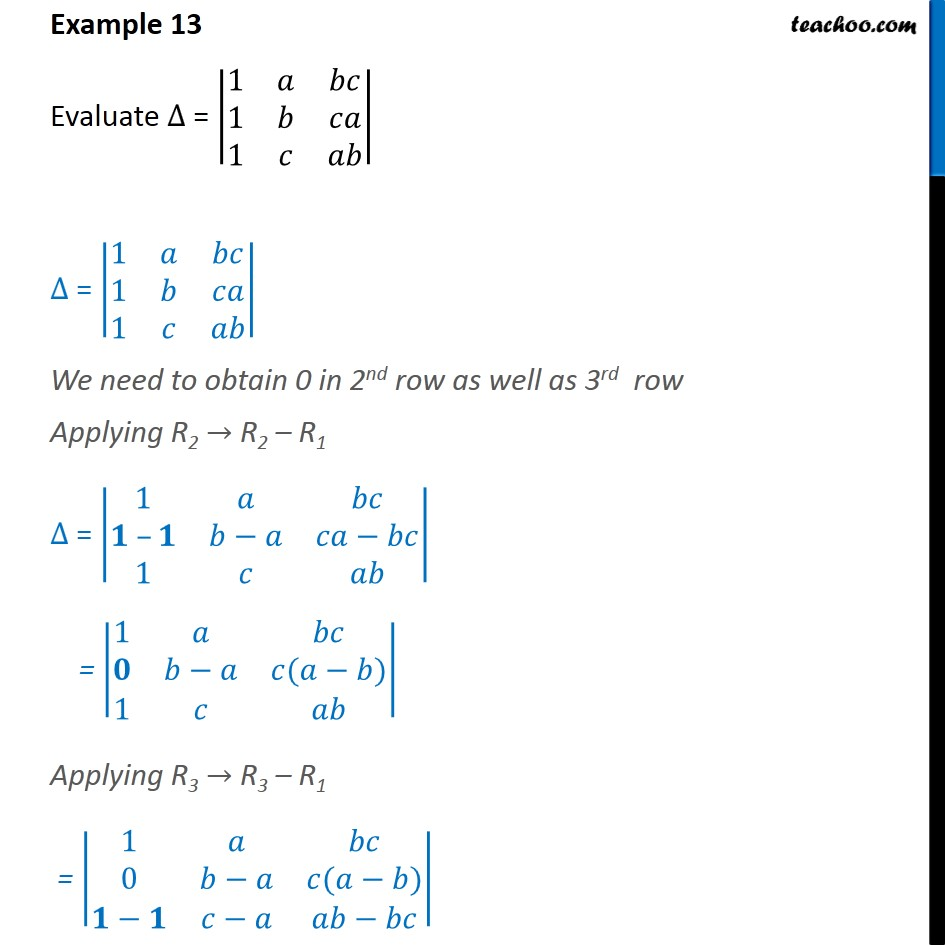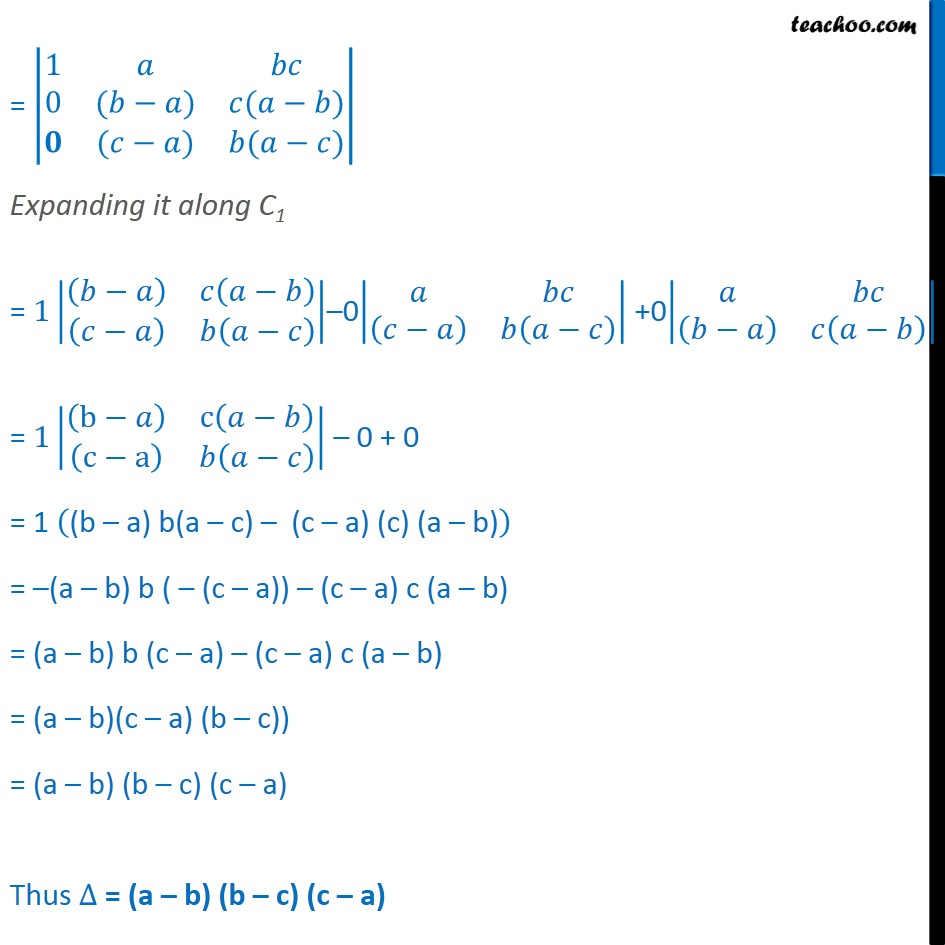Whole row/column one

Chapter 4 Class 12 Determinants
Concept wiseLearn in your speed, with individual attention - Teachoo Maths 1-on-1 Class

### Transcript

Question 8 Evaluate ∆ = 1﷮𝑎﷮𝑏𝑐﷮1﷮𝑏﷮𝑐𝑎﷮1﷮𝑐﷮𝑎𝑏﷯﷯ ∆ = 1﷮𝑎﷮𝑏𝑐﷮1﷮𝑏﷮𝑐𝑎﷮1﷮𝑐﷮𝑎𝑏﷯﷯ We need to obtain 0 in 2nd row as well as 3rd row Applying R2 → R2 – R1 ∆ = 1﷮𝑎﷮𝑏𝑐﷮𝟏 –𝟏﷮𝑏−𝑎﷮𝑐𝑎−𝑏𝑐﷮1﷮𝑐﷮𝑎𝑏﷯﷯ = 1﷮𝑎﷮𝑏𝑐﷮𝟎﷮𝑏−𝑎﷮𝑐(𝑎−𝑏)﷮1﷮𝑐﷮𝑎𝑏﷯﷯ Applying R3 → R3 – R1 = 1﷮𝑎﷮𝑏𝑐﷮0﷮𝑏−𝑎﷮𝑐(𝑎−𝑏)﷮𝟏−𝟏﷮𝑐−𝑎﷮𝑎𝑏−𝑏𝑐﷯﷯ = 1﷮𝑎﷮𝑏𝑐﷮0﷮(𝑏−𝑎)﷮𝑐(𝑎−𝑏)﷮𝟎﷮(𝑐−𝑎)﷮𝑏(𝑎−𝑐)﷯﷯ Expanding it along C1 = 1 𝑏−𝑎﷯﷮𝑐 𝑎−𝑏﷯﷮ 𝑐−𝑎﷯﷮𝑏 𝑎−𝑐﷯﷯﷯–0 𝑎﷮𝑏𝑐﷮ 𝑐−𝑎﷯﷮𝑏 𝑎−𝑐﷯﷯﷯ +0 𝑎﷮𝑏𝑐﷮ 𝑏−𝑎﷯﷮𝑐 𝑎−𝑏﷯﷯﷯ = 1 b−𝑎﷯﷮c 𝑎−𝑏﷯﷮ c−a﷯﷮𝑏 𝑎−𝑐﷯﷯﷯ – 0 + 0 = 1 (b – a) b(a – c) – (c – a) (c) (a – b)﷯ = –(a – b) b ( – (c – a)) – (c – a) c (a – b) = (a – b) b (c – a) – (c – a) c (a – b) = (a – b)(c – a) (b – c)) = (a – b) (b – c) (c – a) Thus ∆ = (a – b) (b – c) (c – a)